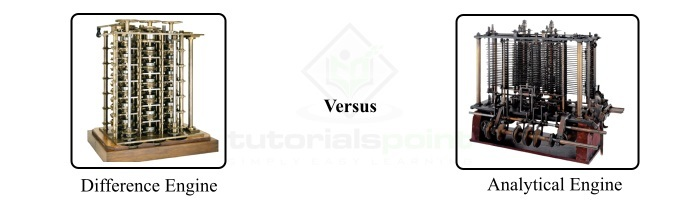# Difference between Analytical Engine and Difference Engine

Difference engine and analytical engine are the two mechanical computing devices invented by the English mathematician Charlese Babbage (Father of Computer) in the 19th century. The fundamental difference between difference engine and analytical engine is that the difference engine can perform basic arithmetic operations like addition, subtraction, multiplication, and division, while the analytical engine can perform more complex operations like trigonometric functions, logarithmic functions, etc. Both difference engine and analytical engine together laid the foundation of modern computing.In this article, we will discuss the important differences between analytical engine and difference engine. But before discussing the differences, let us first discuss their basics.

## What is a Difference Engine?

The difference engine is a mechanical computing machine developed by Charles Babbage in the 19th century. It was designed to calculate mathematical tables that were used in engineering and scientific computations.

The operation of the difference engine was based on the principle of finite differences. Finite differences are a concept in mathematics in which differences are determined between adjacent values in a table containing numbers.

Difference engine use the concept of finite differences to perform basic arithmetic operations such as addition, subtraction, multiplication, and division.

The first prototype of the difference engine consisted of a collection of gears and levers made of brass, and was released in 1822, and it laid the foundation for modern computers.

## What is Analytical Engine?

The analytical engine is also a mechanical computing machine invented by Charles Babbage in the 19th century. The analytical engine was developed to perform complex operations like logarithmic and trigonometric functions, which could not be performed by the difference engine. Overall, the analytical engine was developed to be a general-purpose computing device that can perform any kind of mathematic operations.

The analytical engine makes use of punched cards to manipulate and store data. It consists of a mill that can perform operations like addition, subtraction, multiplication, division, logarithmic operations, and trigonometric functions.

The concept of conditional branching was used in the analytical engine that allows it to perform different calculations based on the results of previous calculations. The analytical engine also supports looping, allowing it to repeat a series of operations multiple times.

After getting insights into the basics of analytical engine and difference engine, let us now discuss all the important differences between them.

## Difference between Analytical Engine and Difference Engine

The important differences between analytical engine and difference engine are listed in the following table:

S.No.

Difference Engine

Analytical Engine

1.

The difference engine is a mechanical computing device that was designed to perform basic arithmetic operations like addition, subtraction, multiplication, and division.

The analytical engine is also a mechanical computing device designed to be a general-purpose machine that could perform any kind of mathematical operations like arithmetic operations, logarithmic operations, trigonometric functions, etc.

2.

Difference engine is considered a simple mechanical calculator.

Analytical engine is considered as a general-purpose computing device.

3.

Difference engine was designed to calculate mathematical tables used in engineering and scientific applications.

Analytical engine was designed to perform any kind of operation used in general-purpose computing.

4.

Difference engine consists of a set of gears and levers made of brass to store and manipulate data.

Analytical engine consists of punched cards to store and manipulate data.

5.

Difference engine was invented in 1822 by the English mathematical Charles Babbage.

Analytical engine was invented in 1837 by Charles Babbage.

6.

7.

Difference engine does not support looping feature.

Analytical engine supports looping feature, allowing it to repeat a series of operations multiple times.

8.

Difference engine does not support conditional branching feature.

Analytical engine supports conditional branching, allowing it to perform different operations based on results of previous calculations.

9.

Difference engine is relatively less complex.

Analytical engine is more complex than difference engine.

10.

Difference engine is less costly than analytical engine.

Analytical engine is more expensive.

## Conclusion

Both difference engine and analytical engine were designed by the English mathematician and inventor, Charlese Babbage in the 19th century. The key difference between difference engine and analytical engine is in the operations performed by them, i.e. the difference engine can only perform basic arithmetic operations like addition, subtraction, multiplication, and division, whereas the analytical engine can perform more complex operations like logarithmic operations and trigonometric operations.

Updated on: 16-May-2023

1K+ Views